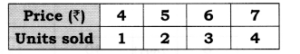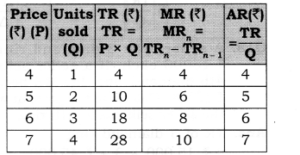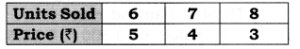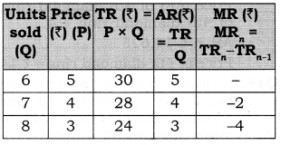# Chapter 8 – Revenue Questions and Answers: NCERT Solutions for Class 12 Micro Economics

Class 12 Introduction to Economics NCERT book solutions for Chapter 8 - Revenue Questions and Answers.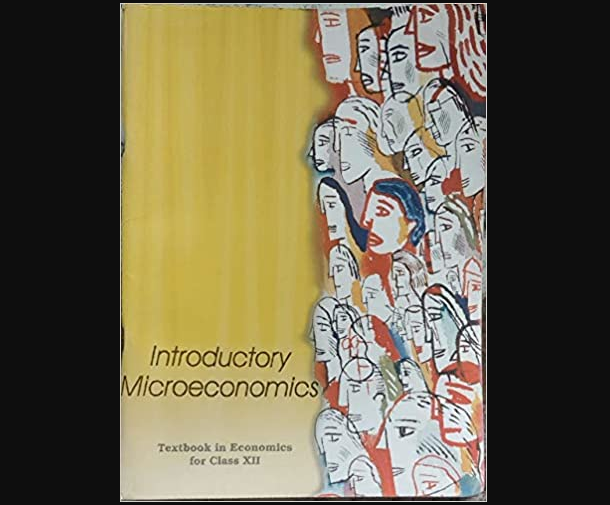## Question 2. Compute the total revenue, marginal revenue and average revenue schedules in the following table. Market price of each unit of good is Rs. 10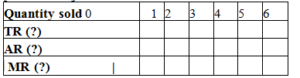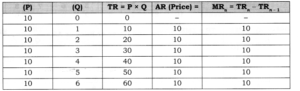## Question 3. What would be the shape of the demand curve so that the total revenue curve is (a) A positively sloped straight line passing through the origin? (b) A horizontal line?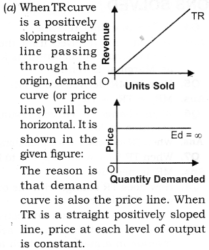### (b) When TR is a horizontal line, demand curve is a rectangular hyperbola. It is shown: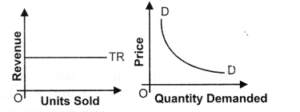## Question 4. Comment on the shape of the MR curve in case the TR curve is a (i) positively sloped straight line, (ii) horizontal straight line. [3-4 Marks]

### Answer: (i) When TR curve is positively sloped straight line, MR is a horizontal line. MR coincides with the demand curve. Price or AR is constant at each level of output.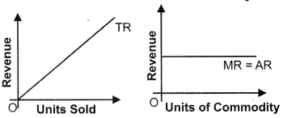### When AR is constant, MR is also constant.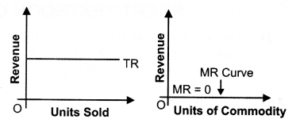## Question 5. From the schedule provided below calculate the total revenue, demand curve and the price elasticity of demand: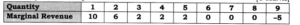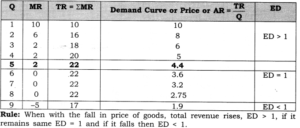## Question 1. Calculate Average Revenue (AR) and Marginal Revenue (MR).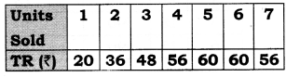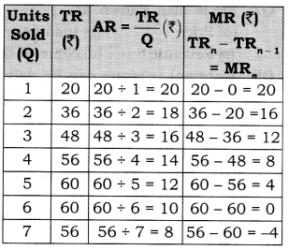## Question 2. Calculate TR and AR from the following data.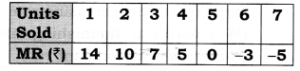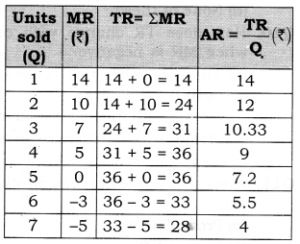## Question 3. Calculate TR and MR from the following data.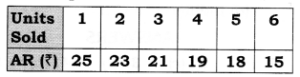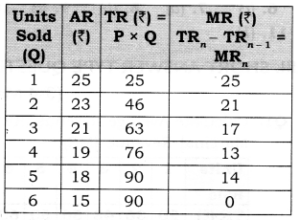## Question 4. Complete the following table.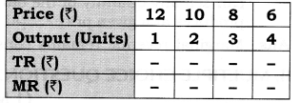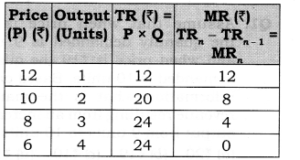## Question 5. Complete the following table.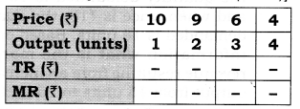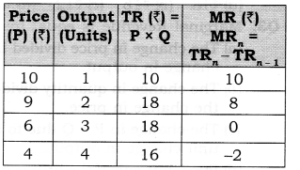## Question 6. Complete the following table.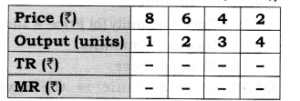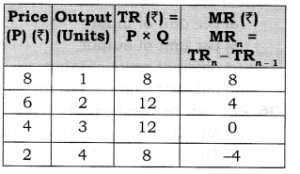## Question 7. Complete the following table.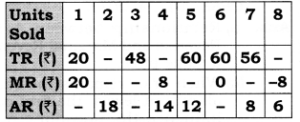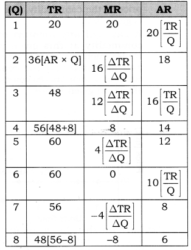## Question 8. Complete the following table.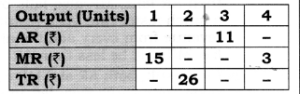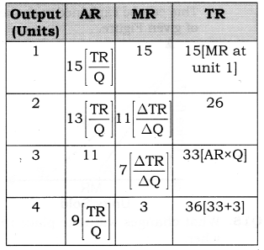## Question 9. Complete the following table.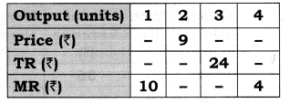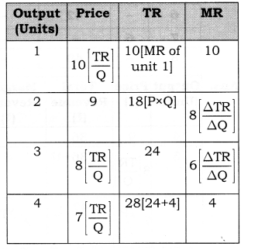## Question 10. Calculate TR, MR and AR.